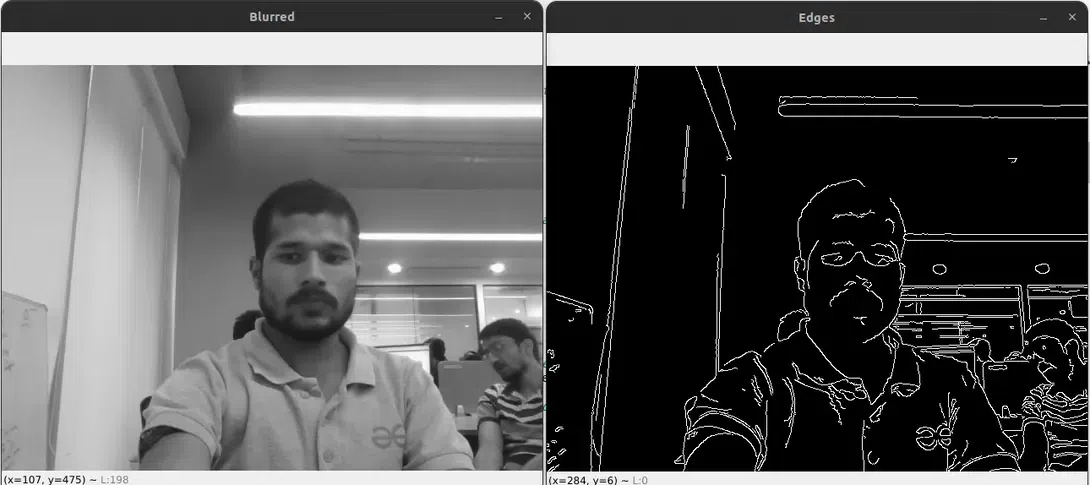# Real-Time Edge Detection using OpenCV in Python | Canny edge detection method

Edge detection is one of the fundamental image-processing tasks used in various Computer Vision tasks to identify the boundary or sharp changes in the pixel intensity. It plays a crucial role in object detection, image segmentation and feature extraction from the image. In Real-time edge detection, the image frame coming from a live webcam or video is continuously captured and each frame is processed by edge detection algorithms which identify and highlight these edges continuously.

In this article, we will use the Canny edge detection algorithms of OpenCV to detect the edges in real-time. It is one of the most widely used edge detection algorithms. Let’s understand the Canny edge detection in depth.

## Canny Edge Detection

Canny Edge Detection is one of the most widely used edge detection algorithms developed by John F. Canny in 1986. It works in four stages i.e Noise reduction, Finding the intensity gradient, Non-maximum suppression, and Hysteresis thresholding.

Let’s understand each stage one by one:

### Step 1: Noise Reduction

First, the noise in the image is reduced by blurring the image. To blur the image we apply the Gaussian filter. It applies a weighted average to each pixel, which reduces the sensitivity with the slight changes in intensity caused by noise. The larger the kernel size, the more significant the smoothing effect. The formula for a 2D Gaussian kernel is given by:### Step 2: Finding the intensity gradient

In this step, the intensity gradient of the image is found, which helps to locate the areas of sudden intensity shifts that correspond to edges in the image. The technique, known as Sobel algorithms are used to compute the first derivative of the image in both the horizontal (Gx) and vertical (Gy) directions. The horizontal gradient (Gx) and vertical gradient (Gy) are given by the following convolution operations:The following equations can be used to calculate the gradient’s intensity (G) and direction (θ) at each pixel:The gradient direction will be always perpendicular to the edges.

### Step 3: Non-maximum Suppression

We use non-maximum suppression to get thin edges. Each pixel is looked at in the gradient direction to see if there is a local maximum. If it isn’t, the pixel is suppressed (its value is set to 0) because it probably isn’t a component of an edge.

### Step 4: Hysteresis Thresholding

Hysteresis thresholding is used to finalize the edges. We set the minVal and maxVal threshold values. Pixels with gradient magnitudes above maxVal are considered strong edges, while those below minVal are considered non-edges and discarded. Pixels with magnitudes between minVal and maxVal are considered weak edges.

To identify the final edges, we follow the strong edges and consider weak edges connected to them as part of the edge. If a weak edge is not connected to any strong edge, it is discarded as noise.

## Python3

 import cv2     def canny_edge_detection(frame):      # Convert the frame to grayscale for edge detection      gray = cv2.cvtColor(frame, cv2.COLOR_BGR2GRAY)             # Apply Gaussian blur to reduce noise and smoothen edges      blurred = cv2.GaussianBlur(src=gray, ksize=(3, 5), sigmaX=0.5)             # Perform Canny edge detection      edges = cv2.Canny(blurred, 70, 135)             return blurred, edges

#### Code explantions

The frame is a single image frame (numpy array) on which we will perform edge detection using the Canny edge detection method.

# Convert the frame to grayscale for edge detectiongray = cv2.cvtColor(frame, cv2.COLOR_BGR2GRAY)

This line converts the input frame from the BGR colour space to grayscale. The Canny edge detection method works on grayscale images, so we first convert the frame to grayscale using cv2.cvtColor() the function.

# Apply Gaussian blur to reduce noise and smoothen edges blurred = cv2.GaussianBlur(src=gray, ksize=(3, 5), sigmaX=0.5)

This line applies Gaussian blur to the grayscale image. The cv2.GaussianBlur() function helps to reduce noise and smoothens the edges, which is useful for obtaining better edge detection results. The (3, 5) argument represents the size of the Gaussian kernel used for blurring and 0 indicates the standard deviation in the X and Y directions.

# Perform Canny edge detectionedges = cv2.Canny(blurred, 70, 135)

This line performs the actual Canny edge detection on the blurred grayscale image. The cv2.Canny() the function takes three arguments: the input image (blurred), the lower threshold (70), and the upper threshold (135). Pixels with gradient magnitude below the lower threshold are considered not edges, and pixels with gradient magnitude above the upper threshold are considered strong edges. Pixels with gradient magnitude between the two thresholds are considered weak edges.

## Python3

 def main():      # Open the default webcam       cap = cv2.VideoCapture(0)             while True:          # Read a frame from the webcam          ret, frame = cap.read()          if not ret:              print('Image not captured')              break                    # Perform Canny edge detection on the frame          blurred, edges = canny_edge_detection(frame)                     # Display the original frame and the edge-detected frame          #cv2.imshow("Original", frame)          cv2.imshow("Blurred", blurred)          cv2.imshow("Edges", edges)                     # Exit the loop when 'q' key is pressed          if cv2.waitKey(1) & 0xFF == ord('q'):              break            # Release the webcam and close the windows      cap.release()      cv2.destroyAllWindows()

## Python3

 if __name__ == "__main__":      main()

Output:### Conclusion

The Canny Edge Detection algorithm provides accurate and robust edge detection by suppressing noise, finding the gradient magnitude and direction, and applying hysteresis thresholding. It has wide applications in computer vision tasks such as object detection, image segmentation, and feature extraction. By implementing this algorithm with the OpenCV library in Python, we can achieve real-time edge detection from live video feeds or webcam streams, enabling us to build various computer vision applications.

Whether you're preparing for your first job interview or aiming to upskill in this ever-evolving tech landscape, GeeksforGeeks Courses are your key to success. We provide top-quality content at affordable prices, all geared towards accelerating your growth in a time-bound manner. Join the millions we've already empowered, and we're here to do the same for you. Don't miss out - check it out now!

Previous
Next